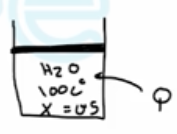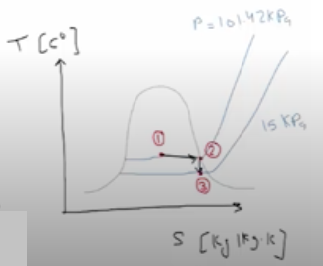Need Help?

Subscribe to Thermodynamics

###### \${selected_topic_name}
• Notes

$\begin{array}{l}{\text { A piston-cylinder device contains } 5 \mathrm{kg} \text { of steam at } 100^{\circ} \mathrm{C} \text { with a quality of } 50 \text { percent. }} \\ {\text { This stam undergoes two processes as follows: }} \\ {1-2 \text { Heat is transferred to the steam in a reversible manner while the temperature is held constant until }} \\ {\text { the steam exists as a saturated vapor. }}\end{array}$

$\begin{array}{l}{\text { 2-3 The steam expands in an adiabatic, reversible process until the pressure is } 15 \mathrm{kPa} \text { . }} \\ {\text { (a) Sketch these processes with respect to the saturation lines on a single T-s diagram. }} \\ {\text { (b) Determine the heat transferred to the steam in process } 1-2, \text { in } \mathrm{kl} \text { . }} \\ {\text { (c) Determine the work done by the steam in process } 2-3, \text { in } \mathrm{kj} \text { . }}\end{array}$$\left.\begin{array}{l}{T_{1}=100 c^{\circ}} \\ {X=0.5}\end{array}\right] \rightarrow h_{1}=h_{f}+x h_{fg}=419.17+0.5(2256.4)=1547.4 kJ/kg$

$\left.\begin{array}{l}{T_{2}=100^{\circ} \mathrm{c}} \\ {\mathrm{x}_{2}=1}\end{array}\right]\rightarrow$$\begin{array}{l}{h_{2}=h_g=2675.6 kJ/kg} \\ {U_{2}=U_g=2506 kJ/kg} \\ {S_2=S_g=7.3540 kJ/kg.k}\end{array}$

$\left.\begin{array}{l}{P_{3}=15 \mathrm{KPa}} \\ {S_{3}=S_{2}=73542}\end{array}\right]\rightarrow U_3=2247.9 kJ/kg$

$E_{i n}-E_{o u t}=\Delta E_{s y s}$

$Q_{i n}-W_{b, out}=\Delta U=m\left(U_{2}- U_{1}\right)$

$Q_{12 \ in} =m\left(h_{2}-h_{1}\right)=5(2675.6-1547,4)$

$∴ Q_{12 \ in} = 5641 kJ$

$W_{23 \ out}=m\left(U_{2} - U_{3}\right)=5(2506-2247.9)=1291 kJ$